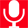Friday, July 10, 2020# Online Entrance Exams

Important Points and Equations

MathematicsApplications of DifferentiationBoolean AlgebraComplex NumbersCo-ordinate GeometryDifferential EquationExponential and Logarithmic SeriesIntegrationPermutations and CombinationsQuadratic EquationsSequence and SeriesBinomial TheoremCirclesConic SectionsDefinite IntegralDifferentiationFunctions, Limit and ContinuityMatrics and DeterminantsProbabilitySetsThree Dimensional Geometry

Information